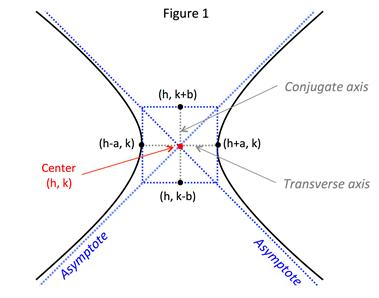# Hyperbola: Asymptotes

Before discussing asymptotes of a hyperbola recall that a hyperbola can have a horizontal or a vertical transverse axis.

Let's quickly review the standard form of the hyperbola.

STANDARD EQUATION OF A HYPERBOLA:

➢ Center coordinates (h, k)

➢ a = distance from vertices to the center

➢ c = distance from foci to center

${c}^{2}={a}^{2}+{b}^{2}\therefore b=\sqrt{{c}^{2}-{a}^{2}}$

$\frac{{\left(x-h\right)}^{2}}{{a}^{2}}-\frac{{\left(y-k\right)}^{2}}{{b}^{2}}=1$transverse axis is horizontal

$\frac{{\left(y-k\right)}^{2}}{{a}^{2}}-\frac{{\left(x-h\right)}^{2}}{{b}^{2}}=1$transverse axis is vertical

A hyperbola has two asymptotes as shown in Figure 1:The asymptotes pass through the center of the hyperbola (h, k) and intersect the vertices of a rectangle with side lengths of 2a and 2b. The line segment of length 2b joining points (h,k + b) and (h,k - b) is called the conjugate axis.

The equations of the asymptotes are:

EQUATION OF THE ASYMPTOTES OF A HYPERBOLA:

➢ Center coordinates (h, k)

➢ a = distance from vertices to the center

➢ c = distance from foci to center

${c}^{2}={a}^{2}+{b}^{2}\therefore b=\sqrt{{c}^{2}-{a}^{2}}$

$y=k±\frac{b}{a}\left(x-h\right)$transverse axis is horizontal

$y=k±\frac{a}{b}\left(x-h\right)$transverse axis is vertical

Let's use these equations in some examples:

Example 1: Find the equations of the asymptotes of the hyperbola
3x2 - 2y2 + 18x + 15 = 0.

 Step 1: Group the x- and y-terms on the left-hand side of the equation. 3x2 - 2y2 + 18x + 15 = 0 $\left(3{x}^{2}+18x\right)+\left(-2{y}^{2}\right)+15=0$ Step 2: Move the constant term to the right-hand side. $\left(3{x}^{2}+18x\right)+\left(-2{y}^{2}\right)=-15$ Step 3: Complete the square for the x- and y-groups. $\left(3{x}^{2}+18x\right)+\left(-2{y}^{2}\right)=-15$ Complete the square for the x-group (3x2 + 18x) Factor out a 3 so the x2-coefficient is 1 3(x2 + 6x) Take the coefficient of the x-term, divide by 2 and square the result. $\frac{6}{2}=3;{3}^{2}=9$ Add the result to the x-group. 3(x2 + 6x + 9) Complete the square for the y-group -2y2 Because there is no y-term no work is necessary. Final result. $3\left({x}^{2}+6x+9\right)-2{y}^{2}=-15+?$ Step 4: Add the values added to the left-hand side to the right-hand side. $3\left({x}^{2}+6x+9\right)-2{y}^{2}=-15+3\left(9\right)$ $3\left({x}^{2}+6x+9\right)-2{y}^{2}=12$ Step 5: Write the x-group and y-group as perfect squares. $3{\left(x+3\right)}^{2}-2{y}^{2}=12$ Step 6: Divide both sides by the value on the right-hand side, simplify and write the denominators as perfect squares. $\frac{3{\left(x+3\right)}^{2}}{12}-\frac{2{y}^{2}}{12}=1$ Divide by 12: $\frac{{\left(x+3\right)}^{2}}{4}-\frac{{y}^{2}}{6}=1$ Write denominators as perfect squares: $\frac{{\left(x+3\right)}^{2}}{{2}^{2}}-\frac{{y}^{2}}{{\sqrt{6}}^{2}}=1$ Step 7: Identify h, k, a and b and the orientation of the transverse axis from the standard equation in Step 6. Center: (h, k) = (-3, 0) Note that x + 3 = x - (-3) a = 2 $b=\sqrt{6}$ The transverse axis is horizontal since x is in the numerator above a2. Step 8: Write the equations of the asymptotes Equation for a horizontal transverse axis: $y=0±\frac{b}{a}\left(x-k\right)$ Substitute: $y=0±\frac{\sqrt{6}}{{2}}\left[x-\left(-{3}\right)\right]$ Simplify: $y=±\frac{\sqrt{6}}{2}\left(x+3\right)$
Example 2: Find the standard equation of a hyperbola having vertices at (4, 3) and (4, 9) and asymptotes $y=4±2x-12$.
 Step 1: Find the center coordinates. Center: The center is the midpoint of the two vertices. Step 2: Determine the orientation of the transverse axis and the distance between the center and the vertices (a). Orientation of the transverse axis: Since both vertices fall on the vertical line x = 4, the transverse axis is vertical. Length of a: To find the length between the center and the vertices take either vertices and find the change in their y-coordinates. Vertex (4, 3): $a=|6-3|=|3|=3$ Vertex (4, 9): $a=|6-9|=|-3|=3$ a = 3 Step 3: Determine the value of b. The given asymptote equation, $y=4±2x-12$ has a slope of 2. Because the transverse axis is vertical, $2=a/b$. $b=\frac{a}{2}=\frac{3}{2}\to {b}{=}{1.5}$ Step 4: Write the standard form of the hyperbola. Equation for a vertical transverse axis: $\frac{{\left(y-k\right)}^{2}}{{a}^{2}}-\frac{{\left(x-h\right)}^{2}}{{b}^{2}}=1$ Substitute: $\frac{{\left(y-{6}\right)}^{2}}{{{3}}^{2}}-\frac{{\left(x-{4}\right)}^{2}}{{\left({1.5}\right)}^{2}}=1$ Final equation: $\frac{{\left(y-6\right)}^{2}}{{3}^{2}}-\frac{{\left(x-4\right)}^{2}}{{\left(1.5\right)}^{2}}=1$

 Related Links: Math algebra Polar Coordinates: Coordinate Conversion Polar Equation: Conversion Between Rectangular Form Pre Calculus

To link to this Hyperbola: Asymptotes page, copy the following code to your site: